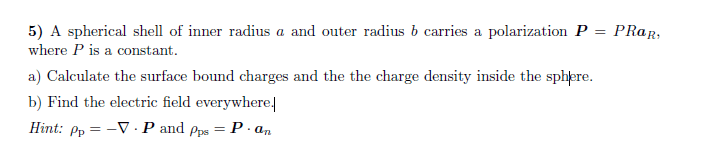# Find the electric field from polarization

• goohu

#### goohu

Homework Statement
See picture.
Relevant Equations
Gauss law for polarizaton : ## -Q = \int P dA ## , dA = element of areaAttempt at solution:
a) Since I need help with b) this section can be skipped. Results :
##ρ_{psa} = -Pa ##
##ρ_{psb} = Pb ##
##ρ_{p} = \frac {-1}{R^2} \frac {∂(R^2PR)}{∂R} = -3P ##

b) This is where I am unsure (first time using gauss law for P) so I need some confirmation here:
## \int E ⋅ ds = \frac {Q} {ε_0} = \frac {1} {ε_0} \int -P dA ##

This will become:
## E(R) 4πR^2 = -\frac {1} {ε_0} PR4πR^2 ##

For 0<R<a : E(R) = 0
For a<R<b : ## E(R) = -\frac {1} {ε_0} PR ##
For b<R : E(R) = 0

The problem here is the solution show : For a<R<b : ## E(R) = -\frac {1} {ε_0} P ##
Did I go wrong somewhere or is this a typo in the solutions?

•